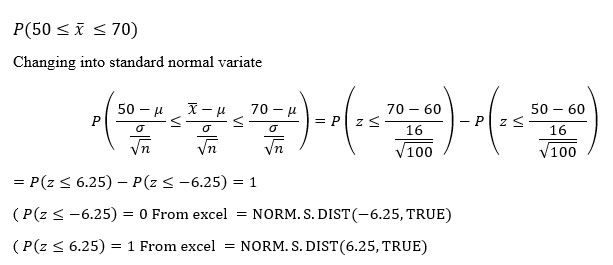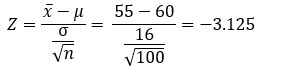# . Sunshine City was designed to attract retired people, and its current population of 50,000 residents has a mean age of 60 years and a standard deviation of 16 years. The distribution of ages is skewed to the left.(a) What is the distribution of the sample mean age of residents for a random sample of 100 residents?(b) What is the probability that a random sample of 100 residents have a mean age between 50 and 70 years of age?(c) What is the probability that the mean age of a random sample of 100 residents is less than 55?

Question
54 views

. Sunshine City was designed to attract retired people, and its current population of 50,000 residents has a mean age of 60 years and a standard deviation of 16 years. The distribution of ages is skewed to the left.
(a) What is the distribution of the sample mean age of residents for a random sample of 100 residents?

(b) What is the probability that a random sample of 100 residents have a mean age between 50 and 70 years of age?

(c) What is the probability that the mean age of a random sample of 100 residents is less than 55?

check_circle

Step 1

6)

Given data

Mean (µ) = 60 years

Standard Deviation(σ) = 16 years

Sample size (n)= 100

a)

As the sample size n > 30

As per central limit theorem, the distribution will be Normal Distribution

b)

probability that a random sample of 100 residents have a mean age between 50 and 70 years of age is given byStep 2

c)

probability that the mean age of a random sample of 100 residents is less than 55 is given by

z-score is given by the following formulaP(X<55) = P(Z<-3.125) = 0.000889 (From Excel =NORM.S.DIST(-3.125,TRUE)

...

### Want to see the full answer?

See Solution

#### Want to see this answer and more?

Solutions are written by subject experts who are available 24/7. Questions are typically answered within 1 hour.*

See Solution
*Response times may vary by subject and question.
Tagged in